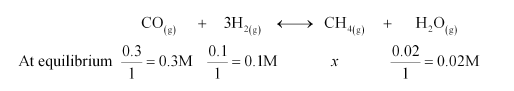# The reaction, CO(g) + 3H2(g)CH4(g) + H2O(g) is at equilibrium at 1300 K in a 1L flask.Question:

The reaction, $\mathrm{CO}(\mathrm{g})+3 \mathrm{H}_{2}(\mathrm{~g}) \longleftrightarrow \mathrm{CH}_{4}(\mathrm{~g})+\mathrm{H}_{2} \mathrm{O}(\mathrm{g})$ is at equilibrium at $1300 \mathrm{~K}$ in a $1 \mathrm{~L}$ flask. It also contain $0.30 \mathrm{~mol}$ of $\mathrm{CO}, 0.10 \mathrm{~mol}$ of $\mathrm{H}_{2}$ and $0.02 \mathrm{~mol}$ of $\mathrm{H}_{2} \mathrm{O}$ and an unknown amount of $\mathrm{CH}_{4}$ in the flask. Determine the concentration of $\mathrm{CH}_{4}$ in the mixture. The equilibrium constant, $\mathrm{K}_{c}$ for the reaction at the given temperature is $3.90$.

Solution:

Let the concentration of methane at equilibrium be x.It is given that $K_{c}=3.90$.

Therefore,

$\frac{\left[\mathrm{CH}_{4(\mathrm{~g})}\right]\left[\mathrm{H}_{2} \mathrm{O}_{(\mathrm{g})}\right]}{\left[\mathrm{CO}_{(\mathrm{g})}\right]\left[\mathrm{H}_{2(\mathrm{~g})}\right]^{3}}=K_{c}$

$\Rightarrow \frac{x \times 0.02}{0.3 \times(0.1)^{3}}=3.90$

$\Rightarrow x=\frac{3.90 \times 0.3 \times(0.1)^{3}}{0.02}$

$=\frac{0.00117}{0.02}$

$=0.0585 \mathrm{M}$

$=5.85 \times 10^{-2} \mathrm{M}$

Hence, the concentration of $\mathrm{CH}_{4}$ at equilibrium is $5.85 \times 10^{-2} \mathrm{M}$.